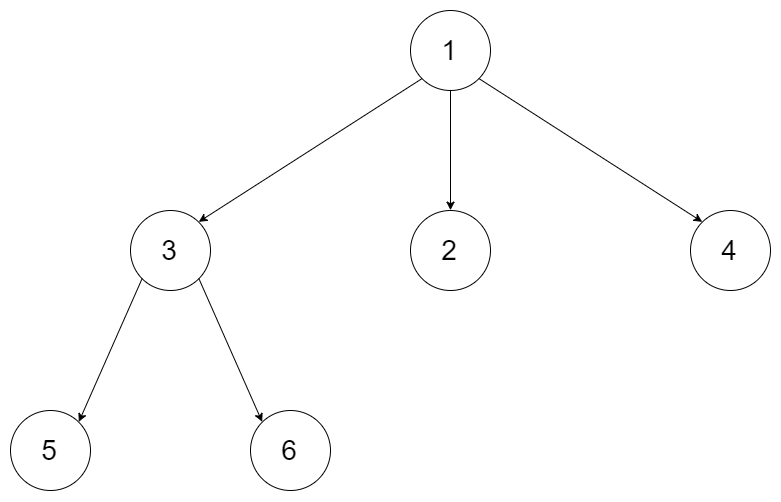559. Maximum Depth of N-ary Tree

#### QUESTION:

Given a n-ary tree, find its maximum depth.

The maximum depth is the number of nodes along the longest path from the root node down to the farthest leaf node.

For example, given a `3-ary` tree:We should return its max depth, which is 3.

Note:

1. The depth of the tree is at most `1000`.
2. The total number of nodes is at most `5000`.

#### SOLUTION:

``````class Solution {
int maxDepthResult = 1;

public int maxDepth(Node root) {
if(root==null) return 0;
for(int i = 0;i<root.children.size();i++){
maxDepthHelper(root.children.get(i),2);
}
return maxDepthResult;
}

public void maxDepthHelper(Node root,int dep){
maxDepthResult = Math.max(maxDepthResult,dep);
for(int i = 0;i<root.children.size();i++){
maxDepthHelper(root.children.get(i),dep+1);
}
}
}
``````
>